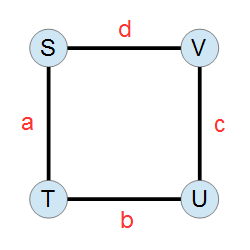## Monday, January 4, 2021

### A Samara week

"Good Bye 2020" was the last round of the year on Codeforces (problems, results, top 5 on the left, analysis). The round has left me with mixed feelings, as I've spent almost the whole 3 hours solving relatively standard problems, and did not have enough time to solve the very exciting problem I. The main reason for that was that I forgot how to count DAGs, and tried to reinvent the wheel in problem H for a very long time. tourist, on the other hand, did not struggle so much with H, and won the round. Well done! Also well done to scott_wu, fivedemands, mnbvmar and qwerty787788 who were able to solve the last problem.

Here's that exciting problem: there is a hidden array of n b-bit integers (in other words, each element is between 0 and 2b-1). You do not know the elements of the array, but you can ask questions about them: in one question, you can ask "is it true that the i-th element of the array is greater than j?" for some value of i between 1 and n and j between 0 and 2b-1. Your goal is to find the value of the maximum element in the array, and you need to do it in O(n+b) queries. The problem actually asked to do it in at most 3*(n+b) queries, but I think just doing it in a linear number of queries is the most interesting part.

Note that simply finding each element with binary search would lead to n*b queries, and it's not clear initially how we can do any better as each query only asks about one element, and the elements are somewhat independent. Can you see the way? n and b are up to 200, and the interactor is adaptive (so your solution most likely needs to be deterministic).

The new year pause was not long, and Open Cup 2020-21 Grand Prix of Samara took place on Sunday (results, top 5 on the left, analysis, overall Open Cup standings). Unlike last year, which saw a fierce competition at the top of the overall Open Cup scoreboard between three teams, this year team USA1 really dominates the proceedings, and they won their 7th Grand Prix out of 8 this time, finishing all problems in 3.5 hours. Congratulations!

The Prime New Year Contest is another staple of the holidays. It is running until the end of this week, and features the problems from 2020 which were not solved by anybody during respective contests. Good luck getting something accepted there, and huge respect to those who already did!In my previous summary, I have mentioned an AtCoder problem: you are given an undirected graph with four vertices and four edges that looks like a square (the picture from the statement on the right). You know that a walk started from vertex S, finished at vertex S, has passed the ST edge (in any direction) a times, the TU edge b times, the VU edge c times, and the SV edge d times. How many different walks fit that description? a, b, c and d are up to 500000, and you need to compute the answer modulo 998244353.

If we replace the ST edge with a parallel edges, the TU edge with b parallel edges, and so on, then we're looking for the number of Euler tours in the resulting graph modulo dividing by some factorials to account for the fact that all parallel edges are equivalent. However, counting Euler tours in undirected graphs is hard.

But given the simple structure of our graph, we can actually reduce our problem to counting Euler tours in directed graphs, which can be done using the BEST theorem! We can iterate over the number of times we pass the ST edge in the direction from S to T, in other words over how many ST arcs would our directed graph have. This determines the number of TS arcs by subtracting from a, then the number of SV and VS arcs from the constraint that the in-degree and out-degree of S must be the same, and so on until we know the number of times we pass each edge in each direction, and we can then count the Euler tours in the resulting graph in constant time (because the graph has 4 vertices and 8 "arc types", and the actual number of parallel arcs does not affect the running time of the BEST theorem). Since a is up to 500000, we have to do this constant time computation 500000 times, which is fast enough.

Thanks for reading, and check back next week!